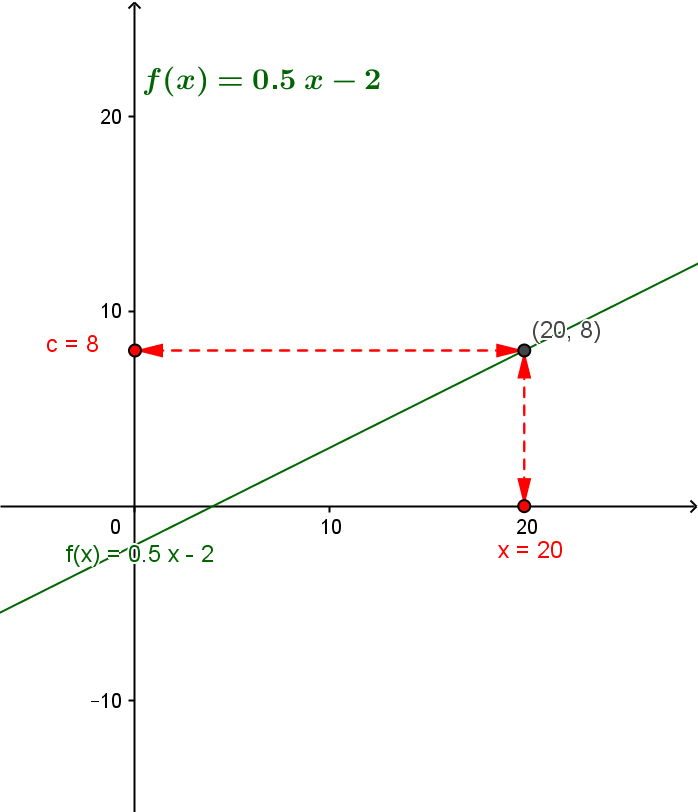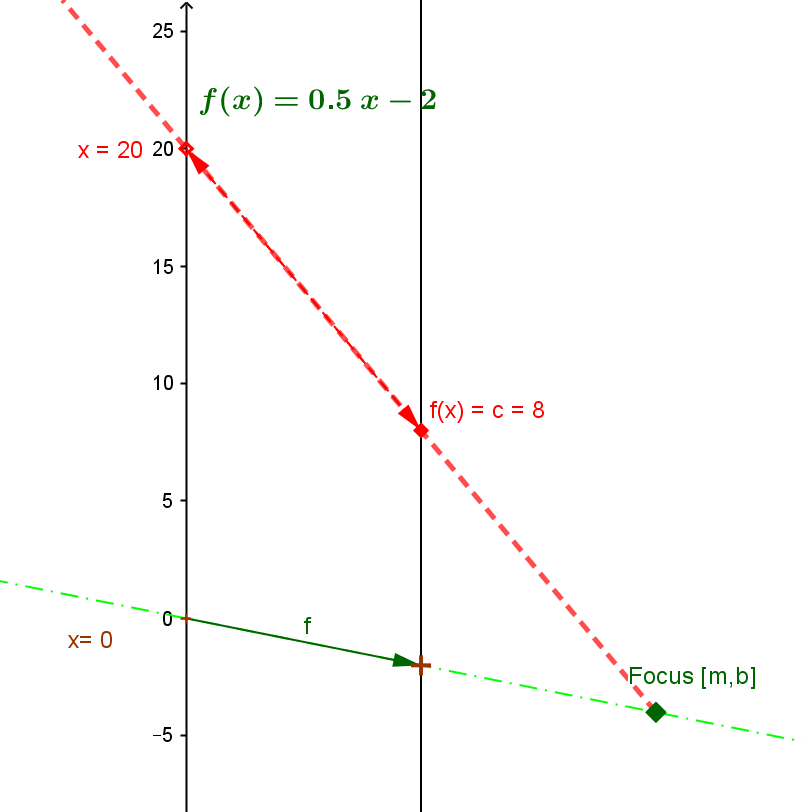Example LF.LEQ.5. Suppose $5x - 10y = 20$ (i) and $y=8$ (ii). Find $x$.
Solution A. Replace $y$ in the equation (i) by $8$ to give the equation $5*x -10*8 = 5x- 80 = 20$ (iii).  This leads to the equation $5x = 100$ (iv) and thus the solution to the solution $x = 20$.
Solution B. Express $y$ as a function of $x$ giving $y = f(x) = \frac 5 {10} x - \frac {20} {10} = \frac 1 2 x - 2$ (v). Now find x where $f(x) = 8 = \frac 1 2 x - 2$, which leads to $x=20$.

In Solution B we find the linear function being used to solve the problem solving a related equation based on the linear function $f$. This solution can be visualized both on the graph and the mapping diagram.

 For the graph of $f$: Find $y=8$ on the Y axis, then find the point on the graph of $f$ directly right (left)  of that point, $(20,8)$, determine it's first coordinate, $20$, and that is the desired value for $x$. For the mapping diagram of $f$: Find $y=8$ on the Y axis , then find the focus point of $f$, $F= [\frac 1 2, -2]$ on the mapping diagram. Draw the line through F and the point $y=8$, to find the point of intersection of this line with the X axis, $x=20$, which is the desired value for $x$.